# Implementation of the Three-Phase Inverter of Medium Power for Applications in Photovoltaic Pumping Systems Avoiding Oversizing

Implementation of the Three-Phase Inverter of Medium Power for Applications in Photovoltaic Pumping Systems Avoiding Oversizing

Luis Mauricio Carrillo-SantosMario Ponce-Silva Yesenia Reyes-Severiano Claudia Cortés-García

Electronic Engineering Department, TecNM/CENIDET, Cuernavaca, Morelos 62490, Mexico

Corresponding Author Email:
mario.ps@cenidet.tecnm.mx
Page:
281-288
|
DOI:
https://doi.org/10.18280/ejee.230401
25 May 2021
|
Accepted:
27 July 2021
|
Published:
31 August 2021
| Citation

OPEN ACCESS

Abstract:

This article presents the development of a three-phase inverter of medium power used in pumping system photovoltaic autonomous, that solves the needs of high consumption of energy in populations away from the national electrical network, whose final application is the use in the agricultural sector. Considering that this application uses an induction motor as a load, it is common to oversize components in such a way that they can withstand the starting current of this, which leads to a negative impact on the volume and cost of the system. This is the reason why it is emphasized the importance of the design with the main objective of selecting the appropriate components for a system, avoiding oversizing. The above is carried out through the implementation of the Sinusoidal Pulse Width Modulation (SPWM) modulation technique operating under the constant torque operation regime. To check the proper operation of the constant torque regime, a comparison with the constant voltage regime is carried out. In addition to the above, the easy implementation of the modulation under the different operating regimes is highlighted by means of the direct link between Matlab/Simulink® and the NUCLEO STM32F767 microcontroller.

Keywords:

modulation technique, switching signals, starting current, induction motor operation mode, photovoltaic system, inverter

1. Introduction

Currently, there is a growing interest in increasing electricity production through photovoltaic solar energy conversion and at the same time ensuring an efficient conversion process, from the starting point to the final application in different economic sectors .

The agricultural sector is one of the sectors in which photovoltaic energy is used to develop different activities related to agriculture and livestock, such as fuels for mechanized agriculture, water pumping for drinking troughs or irrigation, etc.

This paper focuses on the activity of pumping water with solar energy. These types of systems are classified into two groups: systems that operate with direct current pumps and systems that operate with alternating current pumps.

In direct current photovoltaic pumping systems, the main drawback is the low pumping capacity, because the motor used, in most cases, operates at 12 V and low power for applications [2-4]. In addition to the above, the initial investment and pumping capacity ratio for most applications is not cost-effective, as in many cases the required flow is not achieved with a direct current pump which, in addition to low flow, generates constant maintenance costs [5, 6].

As regards alternating current water pumping photovoltaic systems, they have advantages over direct current systems, such as:

• Increased pumping capacity
• Lower maintenance cost
• A higher range of commercial models

However, in the photovoltaic systems of alternating current pumping, an important component is added, the inverter, this one when operating with motors must be able to withstand the starting current of these, which becomes up to six times the nominal current. That is why most sizing and installation manuals propose to oversize the system, which represents a problem concerning the cost of the system [7-10]. Another drawback related to inverters is that the most commercially available ones range from 500 W to 2 kW, which makes it impossible to use pumps with high-power AC motors and, therefore, generate an intermediate flow rate that is insufficient for most agricultural applications [11-16].

It is important to note that there are different methods to perform the design of inverters applied in photovoltaic pumping. However, 65% are considered oversized, leading to excessive energy consumption in this activity. In the following paragraphs, some of the existing design methods found in the literature are discussed.

In works  the design and implementation of an autonomous photovoltaic system is developed based on the required hydraulic energy, the estimation of the available solar energy and the water needs, but in the end the inverter is oversized because its control causes it to have flow variations and thus causes the starting current to increase significantly. On the other hand, in Ref.  probabilistic techniques are used to detect the appropriate oversizing according to the motor load and the available solar energy. All this once again oversizing the system. In addition, there are occasions as discussed in works  that oversizing is used to avoid losses of the Joule effect, but the latter causes the cost of the system to increase.

Correctly sizing a pumping system brings benefits of reducing electricity consumption, which represents economic savings of up to 18% of its current cost [20, 21]. There are several methodologies focused on the correct calculation of the system when a motor is used as a load, as is the case of the photovoltaic pumping system, which range from complex control techniques to additional circuits to the elementary components of the system. For example, Bravo-Tapia et al.  proposed that the starting current reduction is carried out by using an additional regulator circuit. Integrating extra topology causes the cost to rise, reliability to drop, and system lifetime to suffer. Singh et al.  and Barwar et al.  make use of control techniques such as DTC in combination with current tracking to minimize the starting current, increasing the complexity of the system.

Then, according to what has been discussed so far, the importance of having an adequate management of the parameters involved in the behavior of the system elements and that lead to system benefits is highlighted.

This paper presents a solution using an inverter that meets the needs of high-power consumption (at least 10 kW) so that it can drive medium power pumps used in agricultural applications of at least 10 hp; this so that it can withstand the current transients of the same. All of the above, implementing the constant torque operating regime of the induction motor. Also, the constant voltage regime is developed in order to compare and highlight the correct operation of the first regime. Emphasizing the objective of reducing the load starting current to avoid unnecessary oversizing of the system. In addition, a comparison is made of the advantages of the constant torque operation regime with respect to existing methodologies that avoid oversizing.

The rest of the article is organized as follows: Section 2 describes the elements that make up the system under study, including the three-phase inverter, the modulation technique, and the operating regimes of the load used. This section also includes the implementation of the above-mentioned. Section 3 presents the results obtained experimentally, using different loads under two operating regimes: constant voltage and constant torque. Subsequently, section 4 presents the discussion and analysis of the results obtained experimentally from the two operating regimes. It also presents the comparison between the different existing methodologies that avoid the oversizing of the system when an induction motor is used as a load. Finally, section 5 presents the conclusions obtained from this work.

2. Method

This section describes in detail the elements that make up the system. Starting with the three-phase inverter, followed by the generation of switching signals, including the two induction motor operating regimes belonging to this study, which are: constant voltage operation and constant torque operation.

2.1 Three-phase inverter

In autonomous water pumping photovoltaic systems, inverters are the devices responsible for converting direct current from the CD-CD converter into alternating current, which aims to feed specific loads, in this case, a motor pump.

The design of the three-phase inverter is carried out from the data of the power for which the photovoltaic system is designed, which is 10 kW. Table 1 shows the general design specifications for the inverter. The topology selected for the inverter is the conventional three-phase full-bridge type.

Figure 1 shows the schematic diagram of the three-phase inverter used in this study.

For the selection of the circuit breakers that integrate this topology, parameters such as voltage, current, as well as the power of the load for which this converter is used are taken into consideration. Therefore, the power module FP30R06W1E3 from the manufacturer Infineon®.

The SPWM technique is used to modulate the power switches belonging to this topology, which varies according to the operating speed used, either at a constant voltage or constant torque. To select which of them has the best behavior concerning the motor starting current, since this is decisive when sizing the inverter elements of the photovoltaic pumping system.

Table 1. Inverter design specifications

 Parameter Value Power 10 kW Number of phases 3 Bus voltage CD 320 VCD Output voltage between phases 220 V Frequency switching 3.3 kHz

## 1.png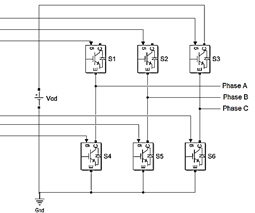Figure 1. Schematic diagram of the three-phase inverter

2.2 Switching signals for three-phase inverter

As shown in the introduction section, strategies have been developed to avoid oversizing. However, they have the disadvantages of control complexity and an increase in the number of power semiconductor devices used. That is why in this paper the design of the system is carried out under the constant torque operation regime. Subsequently, the constant voltage regime is carried out in order to appreciate the correct operation of the first one.

The SPWM modulation technique is used to generate the inverter switching signals, which vary depending on the machine's operating mode.

The SPWM modulation strategy is characterized by a comparison of a carrier signal with reference modulating signals, resulting in switching signals that will activate and deactivate the power switches. Figure 2 shows this comparison, which occurs as follows: when the modulating signal is greater than the carrier signal, then it results in a high pulse (A), and when the modulating signal is less than the modulating signal the results are a low pulse (B).

Table 2 shows the general specifications of the SPWM technique used in this study.

## 2.png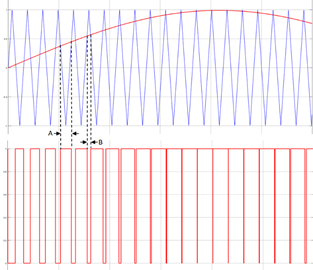Figure 2. Principle of comparison of modulating signal and carrier signal, SPWM modulation strategy

Table 2. SPWM modulation technique specifications

 Parameter Value Modulation signal frequency (Fm) 60 Hz Carrier signal frequency (Fc) 3.3 kHz Modulation index (m) 1 Switching frequency 3.3kHz

2.2.1 SPWM for constant voltage operation

In this mode of operation, the induction motor is supplied at a nominal voltage from the beginning and throughout the time the machine is in operation. Figure 3 shows the schematic diagram with which the switching states that activate and deactivate the power switches of the three-phase topology of the conventional inverter are generated to operate the induction motor at a constant voltage, using the SPWM modulation technique.

## 3.png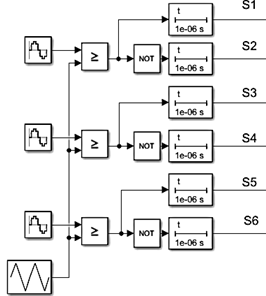Figure 3. Schematic diagram of switching signals for constant voltage operation

The following is a list of the elements that make up the schematic diagram above, belonging to the SPWM modulation stage developed in Matlab Simulink®, as well as the function of each one of them.

- Sine wave generator. This block generates the sine wave or in this case, modulating signal. Being a three-phase system, there are three modulating signals 120° out of phase between them.

- Triangular generator. This block generates the triangular wave, or in this case, carrier signal, whose amplitude is ±1.

- Relational operator. This block compares the modulating and carrier signals. That is, when the modulating signal is greater than the carrier, the output is a high level or 1, and when the modulating signal is lower, the output is a low level or 0.

- NOT logic operator, ≥. This block behaves as a not gate. It is used to obtain the three negated outputs of the complementary switches of the inverter topology.

- Power-on delay. This block delays the output signal by a certain time. This is used because the switching signals between the switches must contain a dead time to avoid an overlap between them.

Emphasizing that the carrier signal and the modulating signals are maintained with the same voltage amplitude during the whole period. That is, throughout the duty cycle are governed by Eqns. (1)-(4).

$V_{T}=A_{T}\left[\frac{2}{\pi} \arcsin \left(\sin \left(2 \pi f_{c} t-\frac{\pi}{2}\right)\right)\right]+A_{T}$     (1)

where: VT is the voltage signal of the triangular carrier. t is the period. fc is the frequency of the carrier signal. AT is the amplitude of the triangular waveform.

$V_{A}=A_{A} \sin \left(2 \pi f_{m} t\right)$     (2)

$V_{B}=A_{B} \sin \left(2 \pi f_{m} t+120^{\circ}\right)$     (3)

$V_{C}=A_{C} \sin \left(2 \pi f_{m} t-120^{\circ}\right)$     (4)

where: VA, VB, and VC are the voltage of each phase A, B, and C, respectively. fm is the modulating frequency of the sine waveform. AA, AB, and AC are the amplitudes of the sine waveform.

2.2.2 SPWM for constant torque operation

This operation regime of the motor is based on the voltage-frequency relation and mainly serves to vary the speed of a motor, varying proportionally the voltage and the frequency maintaining constant the torque of the same one.

The voltage/frequency ratio is obtained by dividing the nominal voltage by the rated frequency of the motor used (see Eq. (5)).

$V F=\frac{V_{n}}{f_{n}}$     (5)

where: Vn is the nominal voltage and fn is the rated frequency of the motor used, respectively.

Once this data has been obtained, it must be ensured that at all times of operation this ratio remains constant, as this ensures that the engine delivers the rated torque at all times. It is important to note that when using speeds between 25% and 100% of the nominal speed of the induction motor used, the magnetic flux of the motor depends only on the voltage-frequency ratio, and also at lower speeds this technique ensures that the motor operates correctly because the induced torque is inversely proportional to the frequency.

To simulate the switching states of this operating mode, first, the nominal voltage and frequency parameters of the system load are taken, in this case, 220 V and 60 Hz, respectively. The reason for taking into account the nominal values of the motor in terms of voltage (220 V) and frequency (60 Hz) is to establish the main voltage-frequency ratio, which must be maintained during the variation steps. In this case, the main variation is 3.66. Therefore, taking into account the above, variation steps are established in such a way that the main voltage-frequency ratio is respected in order to guarantee that the torque remains constant. Starting with reduced voltage and frequency values to contribute to the decrease of the current handled in this step, until reaching the step in which the nominal values of the motor are used.

Table 3 shows the variation steps mentioned above. It can also be seen that the main ratio is respected, varying only ±1%.

Table 3. Voltage-frequency variations

 Time (s) Voltage (V) RMS Frequency (Hz) Voltage-frequency ratio 0 102 28 3.64 15 125 34 3.67 25 145 40 3.62 35 220 60 3.66

Using the data provided in Table 3, the schematic diagram that complies with these characteristics is carried out (Figure 4). The difference between the schematic prepared for the constant voltage regime and the one belonging to this regime lies in the generation of the modulating signals because in this variant these signals are required to be modified throughout the cycle.

## 4.png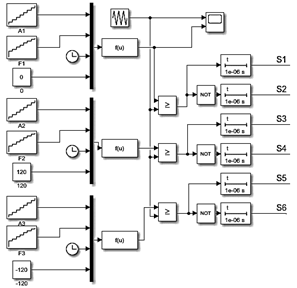Figure 4. Schematic diagram of switching signals for constant torque operation

The blocks used for the generation and modification of the modulating signals belonging to this variant are described below.

- Function generator. This block contains the sine function, which has as inputs the ramp generator blocks, which modify the function in terms of amplitude, frequency and phase.

- Ramp generator. This block modifies the amplitude and frequency of the sine function.

- Constant. This block modifies the phase of the sine function.

Based on what was established in the previous paragraphs, the simulation of the modulating and carrier signals belonging to this regime was carried out. Remembering that the purpose is to compare these signals to obtain the switching states of the three-phase inverter. In this strategy the pulse width varies depending on the amplitude of the sinusoidal modulating wave, which is compared with a triangular carrier wave to determine the switching instants.

Figure 5 shows such a comparison, which occurs as follows: when the modulating signal is greater than the carrier signal, then it results in a high pulse (A), and when the modulating signal is less than the modulating signal, the result is a low pulse (B). Unlike the constant voltage regime, in the constant torque operating regime the modulating signal varies according to the previously established steps (Figure 6). Just as in the same figure, an approach is shown to the carrier and modulating signal.

## 5.png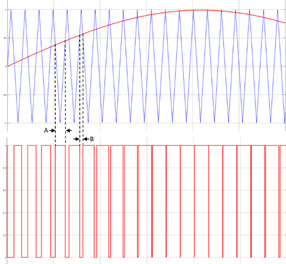Figure 5. Principle of comparison of modulating signal and carrier signal

## 6.png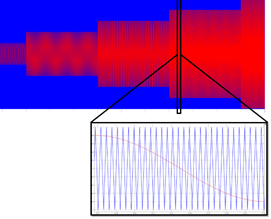Figure 6. Amplitude and frequency variation steps of the modulating signal

The STM32F767® development card was used to implement the switching states, with the collaboration of the Matlab Simulink® program and the embedded code support package for STMicroelectronics® cards. The Matlab Simulink® program's collaboration with Nucleo STM development cards makes it easy to implement prototypes by generating C and C language code from models, which are located within the block library.

Figure 7 shows the switching signals already implemented in the STM32F767 microcontroller. Here you can also see a close-up of a switching signal with its complementary signal, which includes the dead time between them (1us).

## 7.png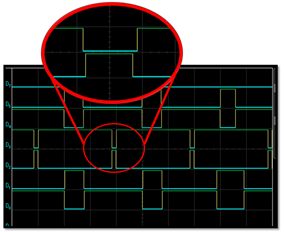Figure 7. Implemented switching signals, with dead times

3. Results

In this section, the results obtained using medium-power three-phase induction motors operating in the two regimens addressed are found. Figure 8 shows the implemented circuit of the three-phase inverter in conjunction with the drive circuits, which amplify the power output from the STM32F67 board. It is important to mention that the use of this specific microcontroller brings with it the advantage of a simple digital implementation, since it is only required to link it with the simulation carried out in MatLab/Simulink® and the programming is done by itself. This avoids creating more lines of extra code, thus reducing the complexity of implementation.

Also, in Figure 9 the voltage waveform at the inverter output obtained with this circuit is shown using the conventional SPWM modulation technique. It shows that the design voltage and frequency are met since it has a period of 16.66 ms, i.e., 60 Hz; and a nominal voltage of 220 VRMS between phases. This is based on the nominal specifications of the engines used in the experimental tests.

## 8.png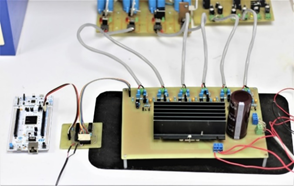Figure 8. Implemented circuit of the three-phase inverter

## 9.pngFigure 9. Voltage waveform at the inverter output

SPWM modulation technique was used in conjunction with a 10 hp induction motor as a system load working at idle, under constant voltage and constant torque operating rates. Table 4 contains the specifications for the induction motor used in the simulation.

As mentioned above, the comparison parameter to be considered between the two operating speeds is the amplitude of the induction motor starting current used as a load, as this represents an important parameter to avoid oversizing the inverter.

The importance of knowing the behavior of the motor starting current under different operating regimes is because during the starting of a motor, the current demanded can be up to 6 times the nominal current, since a large initial power is required to overcome all the resistances from the motor's rest to its final speed.

This high current can cause a voltage drop that affects the operation of the installation or system to which it is attached. In addition, it generates inconveniences of winding heating and thus affects the useful life of the system. Sometimes, when there is no technique for the management of the starting current, it is necessary to resort to the oversizing of elements that belong to the system, in this case the three-phase inverter.

Table 4. General specifications for the 10 hp induction motor

 Parameter Value Nominal power 10 hp Nominal voltage 220 V Nominal frequency 60 Hz Rated speed 1770 rpm No-load current 8.7 A Full load current 28.2 Number of poles 4 Number of phases 3

Figure 10 shows the starting current obtained in the three phases of the 10 hp three-phase induction motor using the SPWM modulation technique for constant voltage. It can be observed that the starting current amplitude becomes 45 A.

Figure 11 shows the starting current in the three phases obtained from the same induction motor but for constant torque. It can be seen that unlike the result obtained with the constant voltage mode, the amplitude of the starting current has a value of 10 A.

## 10.png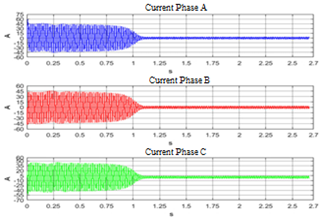Figure 10. 10 hp induction motor starting current using SPWM technique for constant voltage operation

## 11.png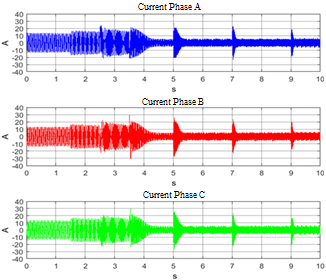Figure 11. 10 hp induction motor starting current using SPWM technique for constant torque operation

Comparing the currents presented in the figures above, it can be observed that there is a considerable difference between the amplitude of the starting current operating in the constant voltage regime and the starting current in the constant torque regime. The difference between these amplitudes is 35 A.

Subsequently, experimental tests were continued on other motors with different powers (1 hp and 3 hp), to corroborate the good performance for medium-power motor pumps.

## 12.png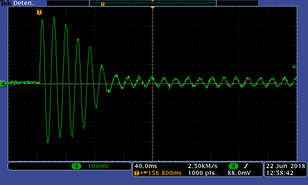Figure 12. Motor starting current of 1 hp, constant voltage operation

## 13.png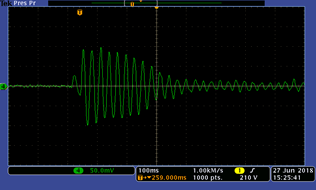Figure 13. Motor starting current of 1 hp, constant torque operation

## 14.png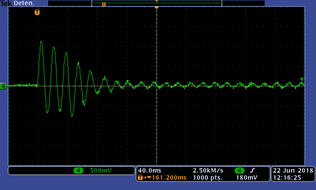Figure 14. Motor starting current of 3 hp, constant voltage operation

## 15.png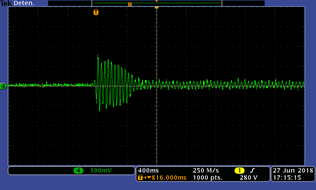Figure 15. Motor starting current of 3 hp, constant torque operation

Figures 12 and 13 show the starting current obtained from the 1 hp motor in constant voltage and constant torque regimes, respectively. Likewise, Figures 14 and 15 show the starting current for the 3 hp induction motor obtained experimentally.

4. Discussion of Results

Analyzing the results obtained experimentally, it is found that the obtained with both motors, as with the 10 hp motor, reflect that the constant torque speed reduces the value of the starting current in the induction motors used. Table 5 shows the comparison of these results concerning the behavior of the starting current amplitude in the induction motors used in this study.

Table 5. Comparison of starting current in the induction motors used

 Induction motor power (hp) Starting current amplitude (A) Constant voltage regime Constant torque regime 1 32 A 9 A 3 113 A 16 A

The percentage of decrease in the amplitude of the starting current at the resulting constant torque regime for the 1 hp induction motor is 71.87% and for the 3 hp induction motor is 85.84%. This represents an advantage because reducing the starting current of the motor contributes to an evident decrease in the size of the components of the photovoltaic system because the total current effort of the system decreases and with it, the total cost of the system is reduced.

Also, lowering the starting current avoids unnecessary oversizing of the system, as this commonly occurs because the starting current can be up to six times the nominal current of medium power induction motors.

Considering the existing methods to avoid oversizing, Table 6 shows a summary of each method studied and their disadvantages. Subsequently, the contributions of implementing the constant torque regime discussed in this article are highlighted.

Table 6. Existing methods to avoid oversizing

 Method used to avoid oversizing Disadvantages  Adds an extra electronic circuit, which serves the function of reducing the starting current. Increased system size and cost. Reduced system life and reliability.  It reconfigures the system in such a way that at the moment of starting the motor, 70% of the energy required by the system is supplied by the grid and only 30% by the photovoltaic system. Requires interconnection to the grid to supply at least 70% of system capacity at motor start-up. [23, 24] Employs DTC control combined with current tracking. Set delay times to split the load and enlarge the PV plant. Increase in the complexity of digital implementation. Increase in system size.

Contrary to what is presented in the previous table, the constant torque regime implemented to decrease the starting current and thus avoid oversizing has the advantage of doing all of the above without increasing the number of power semiconductor devices, supports the total power required by the system without the need of the grid, uses simple control by modifying the SPWM and is easy to implement digitally due to the direct link between the platform and the microcontroller.

5. Conclusions

The conventional three-phase topology is used in this system. The circuit breakers that conform to this topology require modulation techniques to perform their activation and deactivation and thus generate the output wave of alternating current. To do this, the SPWM modulation technique was used, which varied depending on the operating regime used (constant voltage or constant torque).

Emphasizing that one of the design objectives of the inverter is to have the capacity to support medium power loads, avoiding over-dimensioning, it can be said that the SPWM modulation technique at constant torque is appropriate for this application, since it presented a decrease of 77.77%, 71.87%, and 85.84%, in the starting current of the motors analyzed.

Finally, compared to existing strategies that aim to avoid oversizing the system, the constant torque operation regime developed in this paper offers significant advantages, such as simple control, easy digital implementation (by ensuring direct linkage between MatLab SImulink® and the Nucleo® microcontroller without the need for extra codes), avoiding the use of additional system topologies, which minimizes costs, increases system reliability and extends system lifetime.

Nomenclature
 Fm Frequency of the sine waveform Fc Frequency of the carrier signal IM Induction motor m Modulation index PWM Pulse Width Modulation
References

 Coldwell, P.J. (2019). Reporte de avance de energías limpias, Primer Semestre 2018. S. Secretaría de Energía, Ed., ed. México. https://www.gob.mx/cms/uploads/attachment/file/418391/RAEL_Primer_Semestre_2018.pdf., accessed on April 12, 2021.

 Kaddour, H., Dib, A. (2021). Design a new DC-DC converter for a grid connected photovoltaic system. European Journal of Electrical Engineering, 23(1): 79-86. https://doi.org/10.18280/ejee.230110

 Maddalena, E.T., Moraes, C.G.d.S., Bragança, G., Junior, L.G., Godoy, R.B., Pinto, J.O.P. (2019). A battery-less photovoltaic water-pumping system with low decoupling capacitance. IEEE Transactions on Industry Applications, 55: 2263-2271. http://doi.org/10.1109/TIA.2019.2900412

 Elgendy, M.A., Zahawi, B., Atkinson, D.J. (2010). Comparison of directly connected and constant voltage controlled photovoltaic pumping systems. IEEE Transactions on Sustainable Energy, 1: 184-192. http://doi.org/10.1109/TSTE.2010.2052936

 Adjati, A., Rekioua, T., Rekioua, D., Tounzi, A. (2020). Study of dual stator induction motor in photovoltaic-fuel cell hybrid pumping application. Journal Européen des Systèmes Automatisés, 53(5): 601-608. https://doi.org/10.18280/jesa.530502

 Campana, P.E., Li, H., Zhang, J., Zhang, R., Liu, J., Yan, J. (2015). Economic optimization of photovoltaic water pumping systems for irrigation. Energy Conversion and Management, 95: 32-41. https://doi.org/10.1016/j.enconman.2015.01.066

 Adjati, A., Rekioua, T., Rekioua, D. (2021). Use of the dual stator induction machine in photovoltaic - Wind hybrid pumping. Journal Européen des Systèmes Automatisés, 54(1): 115-124. https://doi.org/10.18280/jesa.540113

 Filho, J.R.M.F., Mendes, F.R.F., Sousa, J.R.B., Medeiros, C.M.S., Sousa, I.R. (2018). Photovoltaic Panel Based Pumping System: A Solution Without Batteries. IEEE Latin America Transactions, 16(2): 514-520. https://doi.org/10.1109/TLA.2018.8327407

 Endo, E., Kurokawa, K. (1994). Sizing procedure for photovoltaic systems. Proceedings of 1994 IEEE 1st World Conference on Photovoltaic Energy Conversion - WCPEC (A Joint Conference of PVSC, PVSEC and PSEC), 1: 1196-1199. http://doi.org/10.1109/WCPEC.1994.520178

 Koussaila, I., Lyes, K., Himour, K., Abdelhakim, D., Azeddine, H., Kaci, G., Fouad, B.M. (2020). Impact of polyphase induction motor on photovoltaic water pumping system. Journal Européen des Systèmes Automatisés, 53(6): 763-770. https://doi.org/10.18280/jesa.530602

 Singh, B., Sharma, U., Kumar, S. (2018). Standalone photovoltaic water pumping system using induction motor drive with reduced sensors. IEEE Transactions on Industry Applications, 54(4): 3645-3655. https://doi.org/10.1109/TIA.2018.2825285

 Shankar, V., Kumar, A., Tiwari, A. (2019). Performance analysis of three phase voltage source inverter using PWM and SPWM Techniques. 2019 International Conference on Computing, Power and Communication Technologies (GUCON), pp. 759-763.

 Khare, A., Rangnekar, S. (2014). Optimal sizing of a grid integrated solar photovoltaic system. IET Renewable Power Generation, 8(1): 67-75. http://doi.org/10.1049/iet-rpg.2012.0382

 Shaji, L., Bansal, P., Bhuria, V. (2020). Performance analysis of a new single phase single source 7-level inverter topology using different SPWM techniques. 2020 IEEE First International Conference on Smart Technologies for Power, Energy and Control (STPEC), pp. 1-6. https://doi.org/10.1109/STPEC49749.2020.9297703

 Rao, R.K., Srinivas, P., Kranthikumar, S. (2014). Simulation and analysis of electrical water pumping system using solar energy. 2014 International Conference on Smart Electric Grid (ISEG), pp. 1-6. http://doi.org/10.1109/ISEG.2014.7005605

 Deepa, K., Kumar, P.A., Krishna, V.S., Rao, P.N.K., Mounika, A., Medhini, D. (2017). A study of comparative analysis of different PWM techniques. 2017 International Conference on Smart Technologies for Smart Nation (SmartTechCon), pp. 1144-1149. http://doi.org/10.1109/SmartTechCon.2017.8358548

 Said, W., Gaber, H.A., Mami, A. (2017). Sizing and simulation of an energy sufficient stand-alone PV pumping system. International Conference on Promising Electronic Technologies (ICPET), pp. 8-13. https://doi.org/10.1109/ICPET.2017.8

 Ali, A, Mahmoud, K., Raisz, D., Lehtonen, M. (2021). Probabilistic approach for hosting high PV penetration in distribution systems via optimal oversized inverter with watt-var functions. IEEE Systems Journal, 15(1): 684-693. https://doi.org/10.1109/JSYST.2020.2982467

 Mouli, G.R.C., Bauer, P., Zeman, M. (2016). System design for a solar powered electric vehicle charging station for workplaces. Applied Energy, 168: 434-443. https://doi.org/10.1016/j.apenergy.2016.01.110

 Hajdidj, M.S., Nacereddine, B.T., Faouzi, D. (2017). Study and optimization of a renewable system of small power generation. European Journal of Electrical Engineering, 3: 133-154. https://doi.org/10.3166/EJEE.19.133-154

 Maranhão, G.N.A., Brito, A.U., Pinho, J.T., Fonseca, J.K.S., Leal, A.M., Macêdo, W.N. (2016). Experimental results of a fuzzy controlled variable-speed drive for photovoltaic pumping systems: A Review. IEEE Sensors Journal, 16: 2854-2864. http://doi.org/10.1109/JSEN.2016.2524530

 Bravo-Tapia, J., García-Martínez, E., Ramos-Guzmán, F., Estevez-Martinez, F. (2019). Circuito de arranque suave para un motor monofásico de corriente alterna conectado a un Sistema Fotovoltaico. Revista de Innovación Sistemática, pp. 27-39.

 Singh, R.K., Kumar, A., Naick, B.K. (2018). Optimised solar power fed induction motor drive for water pumping application along with load. International Electrical Engineering Congress (iEECON), pp. 1-4. http://doi.org/10.1109/IEECON.2018.8712192

 Barwar, M.K., Tripathi, P.R., Thakura, P.R. (2018). Boost inverter fed three phase induction motor for off grid PV based water pumping system. Fourth International Conference on Advances in Electrical, Electronics, Information, Communication and Bio-Informatics (AEEICB), pp. 1-5. https://doi.org/10.1109/AEEICB.2018.8480940